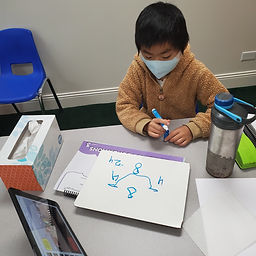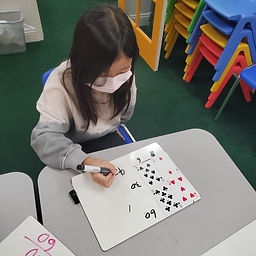## Ms. Raejean

### Target 1​

###### Lesson Type:

Review

Number Operation

:

Computation

Estimate a given quantity based on place-value understandings.

###### 1:

Estimate quantities and use one-to-one correspondence to check the accuracy of the estimate.

###### 2:

Develop a strategy for estimating quantities (i.e., developing a unit of measurement for estimating – if this is what 10 units looks like this must be close to 40 units).

###### 3:

Understand that rounding and estimation are a way to quickly check the accuracy of computations.

###### 4:

Understand that you can use estimation when an exact answer is not needed.

3rd

###### Vocabulary:

Estimation, Estimate

Activities:

• We reviewed the meaning of estimation and estimate. Students estimated about how many marbles were in the images shown. Students referred to previous images to estimate the amount of marbles in subsequent images.
• Students added 2 2-digit numbers. Students used estimation to check the reasonableness of their answers. To estimate, students rounded each number to the nearest ten.### Home Exploration

###### Guiding Questions:## Absent Students:

### Target 2

:

###### 1:

Understand units of measurement and which unit is most appropriate to measure which attribute.

###### 2:

Identify that time can be measured with calendars, clocks, stopwatches, hourglasses, etc.

###### 3:

Identify that weight can be measured with scales, balances, etc.

###### 4:

Identify that capacity can be measured with measuring cups, cylinders, etc.

###### 5:

Identify that length can be measure with rulers, tape measures, etc.

3rd

###### Vocabulary:

Volume, Capacity, Liquid, Weight, Temperature, Height

Activities:

Students identified what attribute each tool measured. Ex. clocks measure time, scales measure weight, etc. Students also identified the appropriate unit of measurement for each attribute. Ex. time is measured in hours and minutes, weight is measured in pounds and ounces.

*We discussed volume - capacity; the amount of space an object takes up.### Home Exploration

###### Guiding Questions:### Target 3

:

###### 1:

Understand that a graph is a visual representation of data.

###### 2:

Become familiar with the vocabulary associated with graphs: unit, scale, title and labels.

###### 3:

Construct and read a scaled bar graph.

###### 4:

Understand that a bar graph can have scaled increments of data.

###### 5:

Understand how to use the scale to accurately read a bar graph.

3rd

###### Vocabulary:

Bar Graph, Scale, X- and Y- axis label, Dozen

Activities:

• Students finished graphing the data from last week. Then we discussed some of the information in the bar graph that was created. Ex. Were there any items for which the same amount was purchased? Which item had the fewest amount purchased? etc.
• Students used data from a table to create a scaled bar graph for which each unit represented 1 dozen eggs.### Home Exploration